# Sentiment analysis using naive bayes classifier

2 de Oct de 2014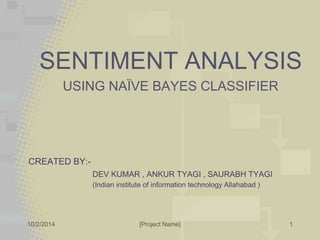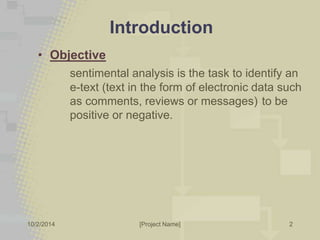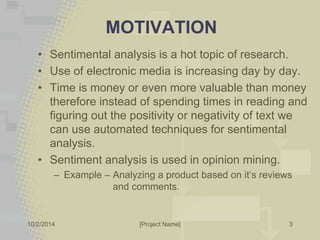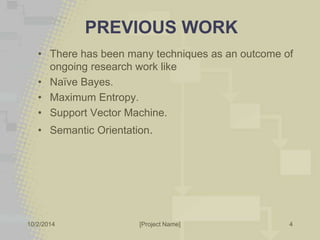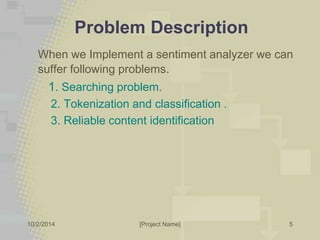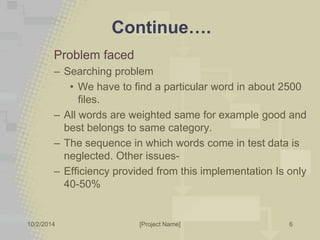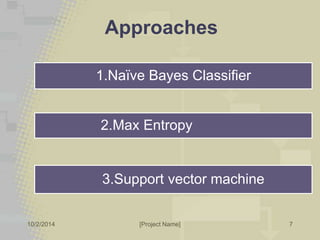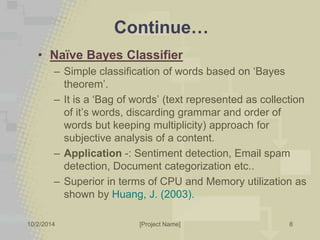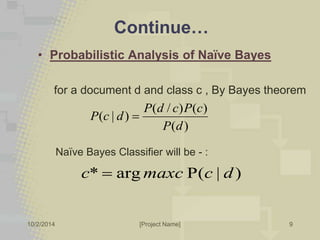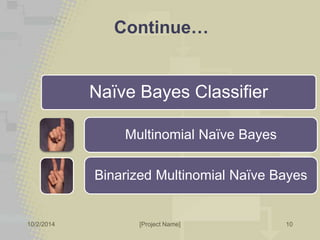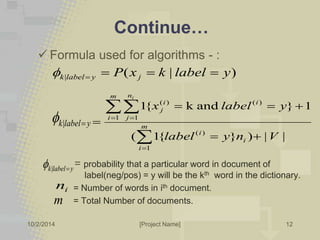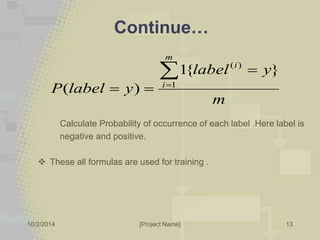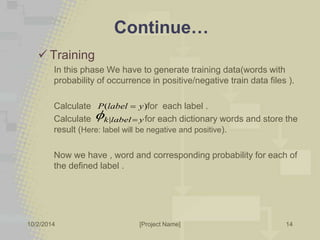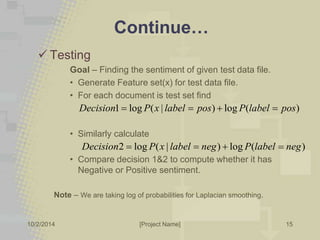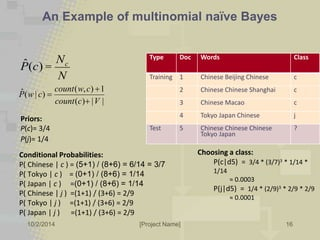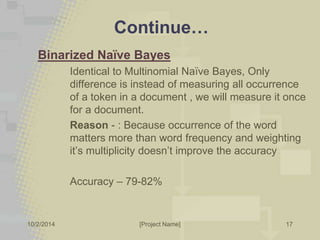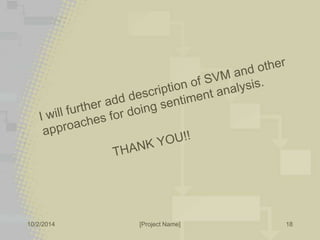1 de 18

### Sentiment analysis using naive bayes classifier

1. SENTIMENT ANALYSIS USING NAÏVE BAYES CLASSIFIER CREATED BY:- DEV KUMAR , ANKUR TYAGI , SAURABH TYAGI (Indian institute of information technology Allahabad ) 10/2/2014 [Project Name] 1
2. Introduction • Objective sentimental analysis is the task to identify an e-text (text in the form of electronic data such as comments, reviews or messages) to be positive or negative. 10/2/2014 [Project Name] 2
3. MOTIVATION • Sentimental analysis is a hot topic of research. • Use of electronic media is increasing day by day. • Time is money or even more valuable than money therefore instead of spending times in reading and figuring out the positivity or negativity of text we can use automated techniques for sentimental analysis. • Sentiment analysis is used in opinion mining. – Example – Analyzing a product based on it’s reviews and comments. 10/2/2014 [Project Name] 3
4. PREVIOUS WORK • There has been many techniques as an outcome of ongoing research work like • Naïve Bayes. • Maximum Entropy. • Support Vector Machine. • Semantic Orientation. 10/2/2014 [Project Name] 4
5. Problem Description When we Implement a sentiment analyzer we can suffer following problems. 1. Searching problem. 2. Tokenization and classification . 3. Reliable content identification 10/2/2014 [Project Name] 5
6. Continue…. Problem faced – Searching problem • We have to find a particular word in about 2500 files. – All words are weighted same for example good and best belongs to same category. – The sequence in which words come in test data is neglected. Other issues- – Efficiency provided from this implementation Is only 40-50% 10/2/2014 [Project Name] 6
7. Approaches 1.Naïve Bayes Classifier 2.Max Entropy 3.Support vector machine 10/2/2014 [Project Name] 7
8. Continue… • Naïve Bayes Classifier – Simple classification of words based on ‘Bayes theorem’. – It is a ‘Bag of words’ (text represented as collection of it’s words, discarding grammar and order of words but keeping multiplicity) approach for subjective analysis of a content. – Application -: Sentiment detection, Email spam detection, Document categorization etc.. – Superior in terms of CPU and Memory utilization as shown by Huang, J. (2003). 10/2/2014 [Project Name] 8
9. Continue… • Probabilistic Analysis of Naïve Bayes for a document d and class c , By Bayes theorem P d c P c ( / ) ( ) Naïve Bayes Classifier will be - : 10/2/2014 [Project Name] 9 ( ) ( | ) P d P c d  c*  argmaxc P(c | d)
10. Continue… 10/2/2014 [Project Name] 10 Naïve Bayes Classifier Multinomial Naïve Bayes Binarized Multinomial Naïve Bayes
11. Continue… Multinomial Naïve Bayes Classifier Accuracy – around 75% Algorithm - :  Dictionary Generation Count occurrence of all word in our whole data set and make a dictionary of some most frequent words.  Feature set Generation - All document is represented as a feature vector over the space of dictionary words. - For each document, keep track of dictionary words along with their number of occurrence in that document. 10/2/2014 [Project Name] 11
12. Continue…  Formula used for algorithms - : ( | ) | P x k label y k label y j      x label y 1{  k and  }  1  k|label y  = probability that a particular word in document of label(neg/pos) = y will be the kth word in the dictionary. = Number of words in ith document. = Total Number of documents. 10/2/2014 [Project Name] 12 ( 1{ } ) | | 1 ( ) 1 1 ( ) ( ) label y n V m i i i m i n j i i j i        k|label y  i n m
13. Continue… i   label y Calculate Probability of occurrence of each label .Here label is negative and positive.  These all formulas are used for training . 10/2/2014 [Project Name] 13 m P label y m i   1 ( ) 1{ } ( )
14. Continue…  Training In this phase We have to generate training data(words with probability of occurrence in positive/negative train data files ). Calculate for each label . Calculate for each dictionary words and store the result (Here: label will be negative and positive). Now we have , word and corresponding probability for each of the defined label . 10/2/2014 [Project Name] 14 P(label  y) k|label y 
15. Continue…  Testing Goal – Finding the sentiment of given test data file. • Generate Feature set(x) for test data file. • For each document is test set find Decision1  log P(x | label  pos)  log P(label  pos) • Similarly calculate Decision2  log P(x | label  neg)  log P(label  neg) • Compare decision 1&2 to compute whether it has Negative or Positive sentiment. Note – We are taking log of probabilities for Laplacian smoothing. 10/2/2014 [Project Name] 15
16. ˆP(c) = Nc N count w c ( , )  1 count c V ( ) | | ˆ ( | ) P w c   Type Doc Words Class Training 1 Chinese Beijing Chinese c Priors: P(c)= 3/4 P(j)= 1/4 Conditional Probabilities: P( Chinese | c ) = (5+1) / (8+6) = 6/14 = 3/7 P( Tokyo | c ) = (0+1) / (8+6) = 1/14 P( Japan | c ) =(0+1) / (8+6) = 1/14 P( Chinese | j ) =(1+1) / (3+6) = 2/9 P( Tokyo | j ) =(1+1) / (3+6) = 2/9 P( Japan | j ) =(1+1) / (3+6) = 2/9 2 Chinese Chinese Shanghai c 3 Chinese Macao c 4 Tokyo Japan Chinese j Test 5 Chinese Chinese Chinese Tokyo Japan Choosing a class: P(c|d5) = 3/4 * (3/7)3 * 1/14 * 1/14 ≈ 0.0003 P(j|d5) = 1/4 * (2/9)3 * 2/9 * 2/9 ≈ 0.0001 10/2/2014 [Project Name] 16 ? An Example of multinomial naïve Bayes
17. Continue… Binarized Naïve Bayes Identical to Multinomial Naïve Bayes, Only difference is instead of measuring all occurrence of a token in a document , we will measure it once for a document. Reason - : Because occurrence of the word matters more than word frequency and weighting it’s multiplicity doesn’t improve the accuracy Accuracy – 79-82% 10/2/2014 [Project Name] 17
18. 10/2/2014 [Project Name] 18